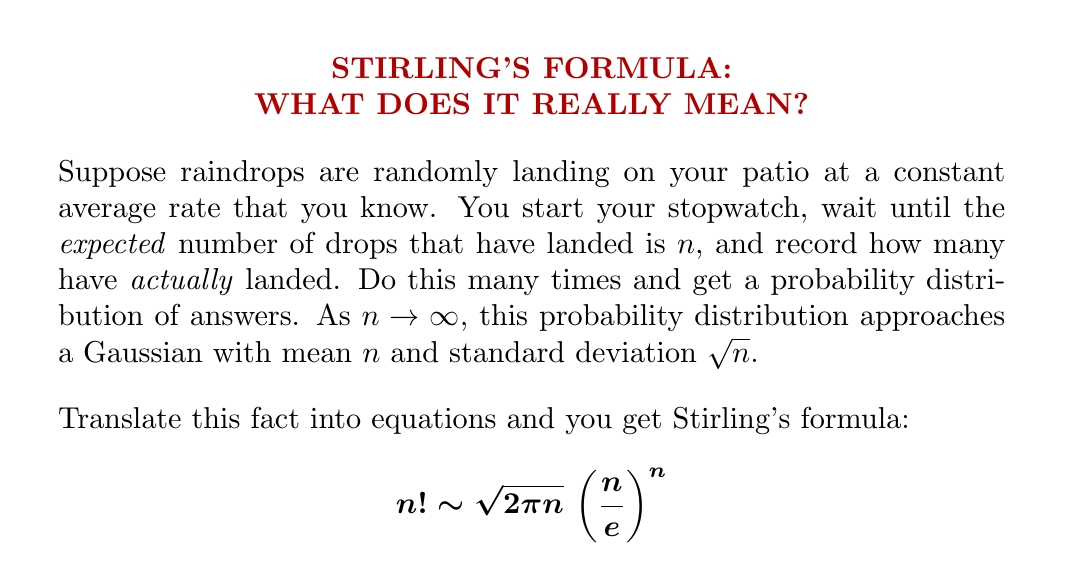## Stirling’s Formula in WordsI recently sketched a quick proof of Stirling’s asymptotic formula for the factorial. But what does this formula really mean?

Above is my favorite explanation. You can’t see the number$2\pi$ in the words here, but it’s hiding in the formula for a Gaussian probability distribution. But what about the factorial, and the rest?

My description in words was informal. I’m really talking about a
Poisson distribution. If raindrops land at an average rate$r,$ this says that after time$t$ the probability of$k$ having landed is$\displaystyle{ \frac{(rt)^k e^{-rt}}{k!} }$

This is where the factorial comes from, and also the number$e.$

Since the average rate of rainfall is$r,$ at time$t$ the expected number of drops that have landed will be$rt.$ Since I said “wait until the expected number of drops that have landed is$n$”, we want$rt = n.$ Then the probability of$k$ having landed is$\displaystyle{ \frac{n^k e^{-n}}{k!} }$

Next, what’s the formula for a Gaussian with mean$n$ and standard deviation$\sqrt{n}$? Written as a function of$k$ it’s$\displaystyle{ \frac{e^{-(k-n)^2/2n}}{\sqrt{2 \pi n}} }$

If this matches the Poisson distribution above in the limit of large$n,$ the two functions must match at the point$k = n,$ at least asymptotically, so$\displaystyle{ \frac{n^n e^{-n}}{n!} \sim \frac{1}{\sqrt{2 \pi n}} }$

And this becomes Stirling’s formula after a tiny bit of algebra!

I learned about this on Twitter: Ilya Razenshtyn showed how to prove Stirling’s formula starting from probability theory this way. But it’s much easier to use his ideas to check that my paragraph in words implies Stirling’s formula, as I’ve just done.

### One Response to Stirling’s Formula in Words

1.Peter Haggstrom says:

John
There is a counterpoint to this. When Paul Levy was doing his foundational work in functional analysis circa 1920 he very simply extracted a Gaussian out of a cosine in the context of working out the volume of a sphere in n dimensions. This fundamental observation was later used by Wiener in his foundational Differential Space paper which dealt with Brownian motion and Wiener gave Levy the credit for the inspiration. I explain what Levy did here: https://gotohaggstrom.com/Extracting%20the%20Gaussian%20from%20integrals%20of%20cosines.pdf
To my knowledge – and for reasons that escape me – his works have never been translated into English. Some years ago I emailed a famous French mathematician and asked him why Levy had been treated so shabbily and got “C’est un outrage” as a reply. I’m still none the wiser. My older sister who is an expert French linguist cannot be convinced to do the job!!
Peter

This site uses Akismet to reduce spam. Learn how your comment data is processed.